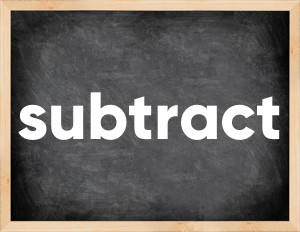# Subtract past tenseThe English verb 'subtract' is pronounced as [səbˈtrækt].
Related to: regular verbs.
3 forms of verb subtract: Infinitive (subtract), Past Simple - (subtracted), Past Participle - (subtracted).

## Here are the past tense forms of the verb subtract

👉 Forms of verb subtract in future and past simple and past participle.
❓ What is the past tense of subtract.

## Subtract: Past, Present, and Participle Forms

Base Form Past Simple Past Participle
subtract [səbˈtrækt]

subtracted [səbˈtræktəd]

subtracted [səbˈtræktəd]

## What are the 2nd and 3rd forms of the verb subtract?

🎓 What are the past simple, future simple, present perfect, past perfect, and future perfect forms of the base form (infinitive) 'subtract'?

### Learn the three forms of the English verb 'subtract'

• the first form (V1) is 'subtract' used in present simple and future simple tenses.
• the second form (V2) is 'subtracted' used in past simple tense.
• the third form (V3) is 'subtracted' used in present perfect and past perfect tenses.

## What are the past tense and past participle of subtract?

The past tense and past participle of subtract are: subtract in past simple is subtracted, and past participle is subtracted.

### What is the past tense of subtract?

The past tense of the verb "subtract" is "subtracted", and the past participle is "subtracted".

### Verb Tenses

Past simple — subtract in past simple subtracted (V2).
Future simple — subtract in future simple is subtract (will + V1).
Present Perfect — subtract in present perfect tense is subtracted (have/has + V3).
Past Perfect — subtract in past perfect tense is subtracted (had + V3).

### subtract regular or irregular verb?

👉 Is 'subtract' a regular or irregular verb? The verb 'subtract' is regular verb.

## Examples of Verb subtract in Sentences

•   - If you award my defendant a fine, I ask that the cost of washing the sheets that the plaintiff stained with her shoes be subtracted from that amount. (Present Simple)
•   Thus, the asthenic builders can subtract 2-3% from the result obtained from the table. (Present Simple)
•   If you subtract salespeople's salaries and other expenses from this amount, you don't get very much. (Present Simple)
•   From this number we must subtract 939,700 servicemen who were reported missing, but after the liberation of the respective territories were newly enlisted in the army. (Present Simple)
•   Otherwise, the owner will subtract the value of the overdue and unsold goods from the seller's wages. (Future Simple)
•   After all, the tax inspectorate, checking on a competitor, easily accesses its counterparties, conducts counter audits and subtracts documents relating to transactions with the company selected for the attack. (Present Simple)
•   Banks, in order to pay customers, began to subtract funds invested in various businesses and industries, financial panic turned into an economic crisis. (Past Simple)
•   - How many miracles were told about the relics, and when churches began to subtract valuables in favor of the starving, nothing was found in these relics. (Past Simple)
•   If the state subtracts land to build a school and pays for the land, that seizure is not a tax. (Present Simple)
•   And we partially started subtracting weapons. (Past Simple)

Along with subtract, words are popular turn off and knock off.

Verbs by letter: , , , , , , , , , , , , , , , , , , , , , , , , .